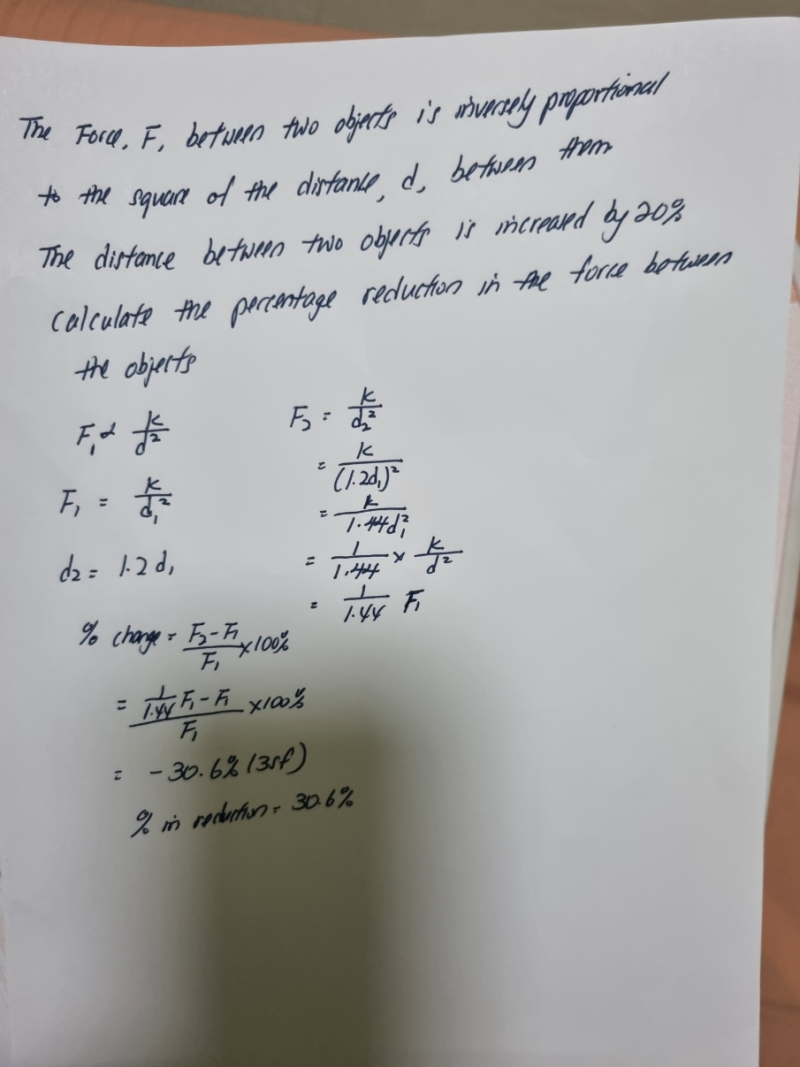# QuestionFor this question, is there any methods to solve as this method is very complicated.pls help thanks.

F = (k/d2 )

Increase in distance by 20% => d =12/10    => New Force = (k/d2 )(10/12)2

ΔF  = Original force – New force

= (k/d2 ) – (k/d2 )(10/12)2

=(k/d2 ) [ 1 – 100/144]

= 44/144  (k/d2 )

Hence % reduction in Force =  44/144 x 100% = 30.6%  (correct to 3sf)

0 Replies 1 Like ✔Accepted Answer